# Volume of a CylinderPage 1

#### WATCH ALL SLIDES

Slide 1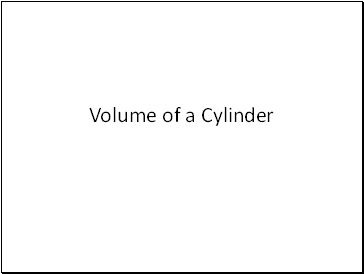Volume of a Cylinder

Slide 2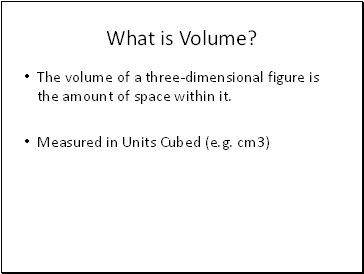## What is Volume?

The volume of a three-dimensional figure is the amount of space within it.

Measured in Units Cubed (e.g. cm3)

Slide 3## Volume of a Prism

Volume of a Prism is calculated by

Volume = Area of cross section x perpendicular height

V = Ah

V = (4 x 4) x 4 = 64 m3

Slide 4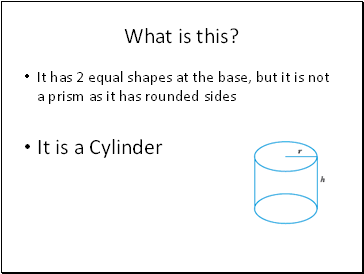What is this?

It has 2 equal shapes at the base, but it is not a prism as it has rounded sides

It is a Cylinder

Slide 5## Volume of a Cylinder

How might we find the Volume of a Cylinder?

Slide 6Example

V = Ah

Slide 7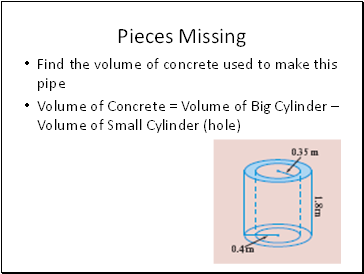## Pieces Missing

Find the volume of concrete used to make this pipe

Volume of Concrete = Volume of Big Cylinder – Volume of Small Cylinder (hole)

Slide 8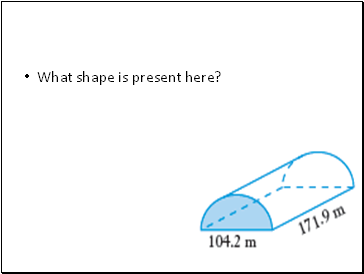What shape is present here?

Slide 9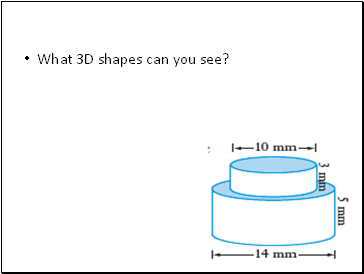What 3D shapes can you see?

Slide 10HOME WORK

Find the Volume of the Solid. To 1 decimal place

Slide 11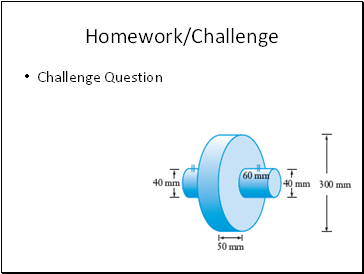Homework/Challenge

Challenge Question

Slide 12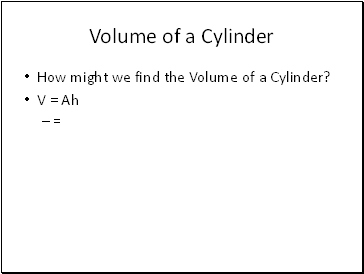Volume of a Cylinder

How might we find the Volume of a Cylinder?

V = Ah

=

Slide 13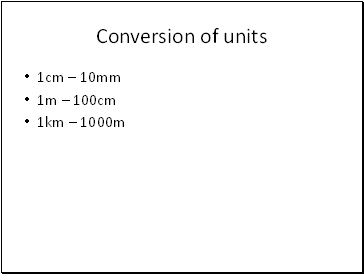## Conversion of units

1cm – 10mm

1m – 100cm

1km – 1000m

Slide 14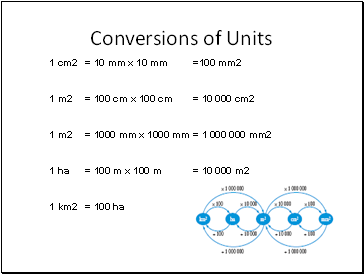Conversions of Units

1 cm2 = 10 mm x 10 mm =100 mm2

1 m2 = 100 cm x 100 cm = 10 000 cm2

1 m2 = 1000 mm x 1000 mm = 1 000 000 mm2

1 ha = 100 m x 100 m = 10 000 m2

1 km2 = 100 ha

Slide 15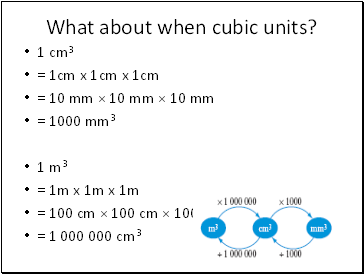## What about when cubic units?

1 cm3

= 1cm x 1cm x 1cm

= 10 mm × 10 mm × 10 mm

= 1000 mm3

1 m3

= 1m x 1m x 1m

= 100 cm × 100 cm × 100 cm

= 1 000 000 cm3

Go to page:
1  2